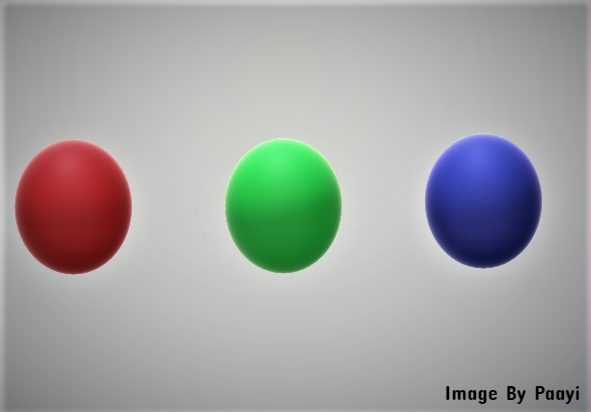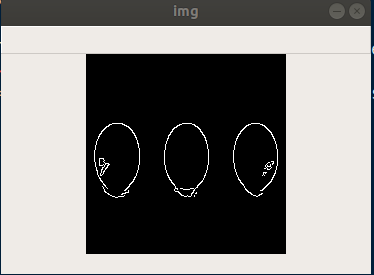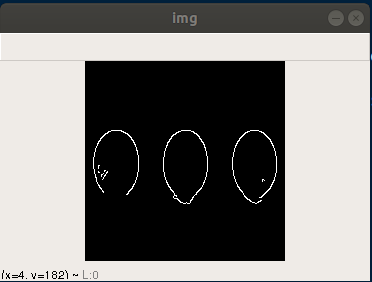## Filtering Techniques with Python OpenCv

##### In this part of Learning Python we Cover Filtering Techniques In Python
Written by |29-Apr-2019 | 0 Comments | 304 Views

Dilation:

It is the opposite of erosion. Just like in erosion where the kernel window moves from one place to the other and erode the boundary away similarly in dilation process the kernel window moves and make the boundary thicker which ultimately dilate the object and increase the area of an object.

The image that we are going to use is as follows:Figure 1

Now we will dilate the image and increase the area of an image with the help of following code:

`import numpy as np`
`import cv2`

`img = cv2.imread('m1.png',0)`
`kernel = np.ones((5,5),np.uint8)`
`dialation = cv2.dilate(img,kernel,iterations = 3)`
`cv2.imshow("img",dialation)`
`cv2.waitKey(0)`

After importing the image, we use the dilate function of OpenCV and dilate the image. The image after the dilation process looks as follows:Figure 2

We can see the image area increases.

Opening:

The opening is another form of filtering technique which is used to remove or reduce the noise from an image. Sometimes we have an image in which there are small contours which cause difficulty. So, to remove such contours, we use opening. In this process, the first image is applied to the process of erosion and then subjected to dilation. In erosion, the particles are first removed. In this process, the boundary of the main object is also somehow affected after the dilation is applied to restore the main object which was affected by the erosion.

So, the image we are going to use is as follows:Figure 3

In Figure 3 we can see that there is a lot of noise which can cause many difficulties if we make contours out of it. These little dots will be considered a separate object. So, to avoid this, we will use the following filter:

`import numpy as np`
`import cv2`

`img = cv2.imread('m2.png',0)`
`kernel = np.ones((5,5),np.uint8)`
`opening = cv2.morphologyEx(img, cv2.MORPH_OPEN, kernel)`
`cv2.imshow("img",opening)`
`cv2.waitKey(0)`

In the above code after reading image and initializing the kernel we call a function of morphology, and the parameters that it except is an image, the flag that tells that Open function should be call and the kernel. The image after morphological operation looks like this:Figure 4

We can now see clearly that all the small dots now disappear. In this way, we can reduce the noise of an image. There are other methods to remove the small contours as well which we will learn in coming lectures.

Closing:

Closing is the opposite of the opening. It is used to remove the noise inside the object. This filtering technique is dilated first, and then erosion occurs. The dilation dilates the boundaries and makes the object area significant which cover all the small holes. Moreover, then after dilation erosion occurs hat restore the image into original thickness.

This filtering technique reduces the chances of making contours within the main object.

Suppose we have an image as follows:Figure 5

There are lots of noise within the image so to remove this following filtering technique is to be used:

`import numpy as np`
`import cv2`

`img = cv2.imread('m3.png',0)`
`kernel = np.ones((10,10),np.uint8)`
`opening = cv2.morphologyEx(img, cv2.MORPH_CLOSE, kernel)`
`cv2.imshow("img",opening)`
`cv2.waitKey(0)`

It is to be noted that we have increased the kernel size here because the holes are big so to make it more effective kernel size should be increased. The image after conversion looks as following:Figure 6

In Figure 6 holes are not present anymore.

Edge Detection:

Edge detection is one of the most import aspects of image processing after filtering technique. It helps the user to reduce the number of pixels to process more efficiently and maintains the structure of an image too. In deep learning algorithm like convolutional Neural Network (CNN) these edge detections are performed inside the hidden layers. However, in the machine learning algorithm, we have to complete it by yourself. Following are an algorithm that is used for edge detection.

Canny Edge Detection:

Canny edge detection is a popular edge detection algorithm. It is the basics of edge detections and image recognition. For image detection, one must have to convert the image into edges. The computer doesn’t understand what is in the image. The only thing it understands is what are pixels and what are the edges saying about that image. In convolution neural network the images are subjected to edge detection in hidden layers so that computer can understand the pattern inside.

In OpenCV, it provides functionality for edge detection. It took three parameters. The first one is the object of an image, and the second one is the minimum value and the third is the maximum value. This minimum and maximum value decide the intensity of edges in an image. No, let’s see the code.

The image that we are going to use is as follows:Figure 7

The image has three balls, and our task is to get the edges of this image. The code for getting the edges is as follows:

`import cv2`
`import numpy as np`

`img = cv2.imread('balls.jpg',0)`
`img = cv2.resize(img,(200,200))`
`edges = cv2.Canny(img,90,200)`
`cv2.imshow("img",edges)`
`cv2.waitKey(0)`

The output that is generated from the above code is as follows:Now we can see the edges of the balls. Much of the information has to be discarded. This function is also used to make the image simplify for the trainer. Now the question arises why it is needed.

In today’s world, we have loads of powerful computer which can perform the tasks in a much efficient way. Still, the GPU’s that graphical processing unit sometime took days to classify an image classification model if the dataset is large. So think how people classified in the 2000s when there were no GPU’s. Thus, the answer is that people make the images simple enough before feeding it into a machine learning algorithm or a neural network. So, these techniques are used to make the images as simple as we can.

Now we will see changing the minimum and maximum value and see what the effects are:

`import cv2`
`import numpy as np`

`img = cv2.imread('balls.jpg',0)`
`img = cv2.resize(img,(200,200))`
`edges = cv2.Canny(img,200,250)`
`cv2.imshow("img",edges)`
`cv2.waitKey(0)`

The output can be seen in Figure 8.Figure 8

More noise is reduced after increasing the values.